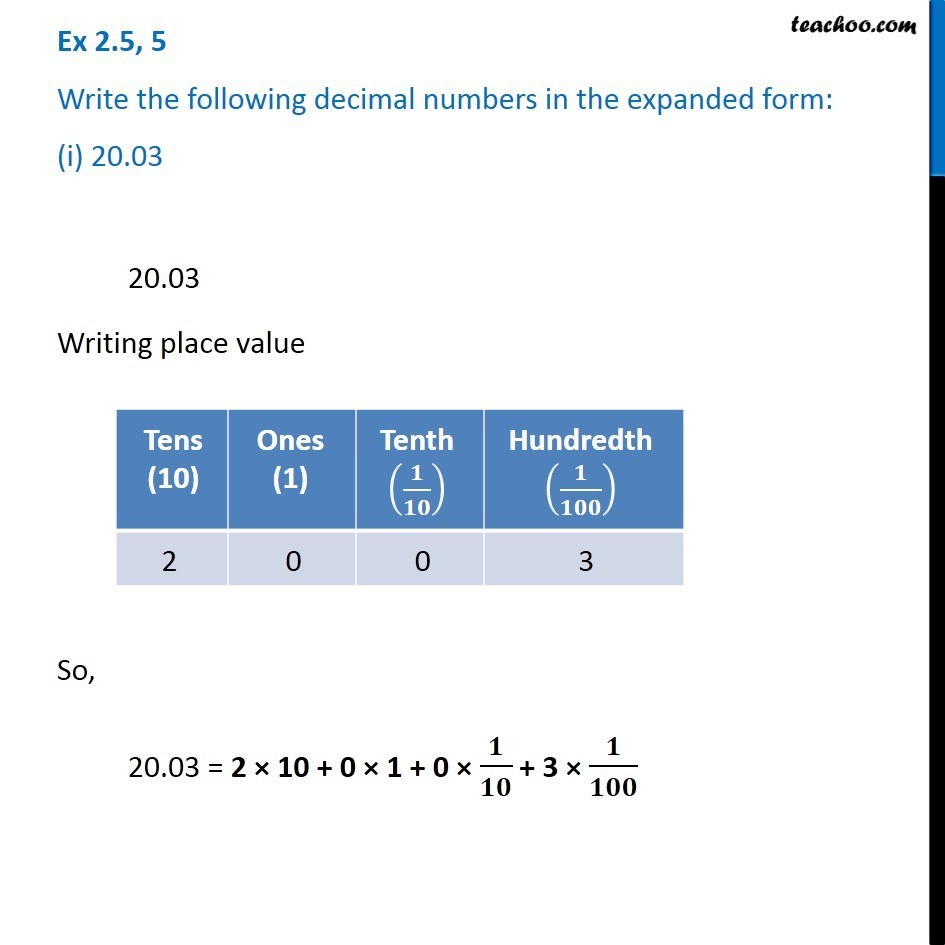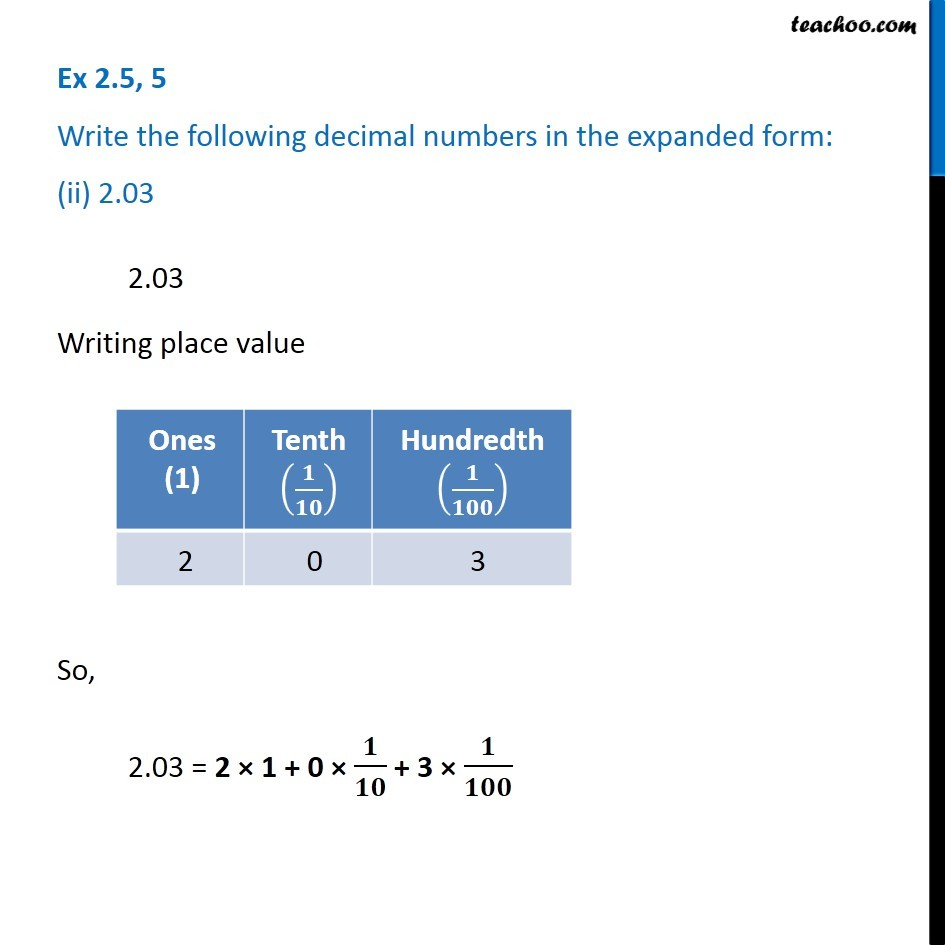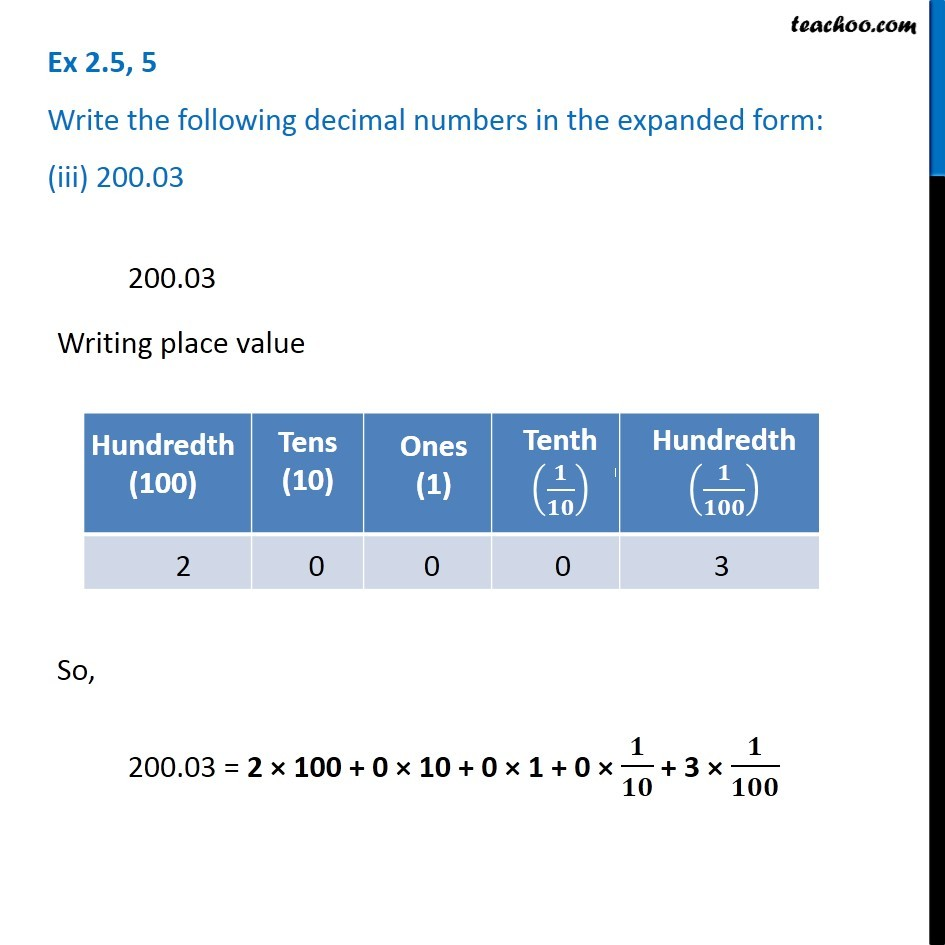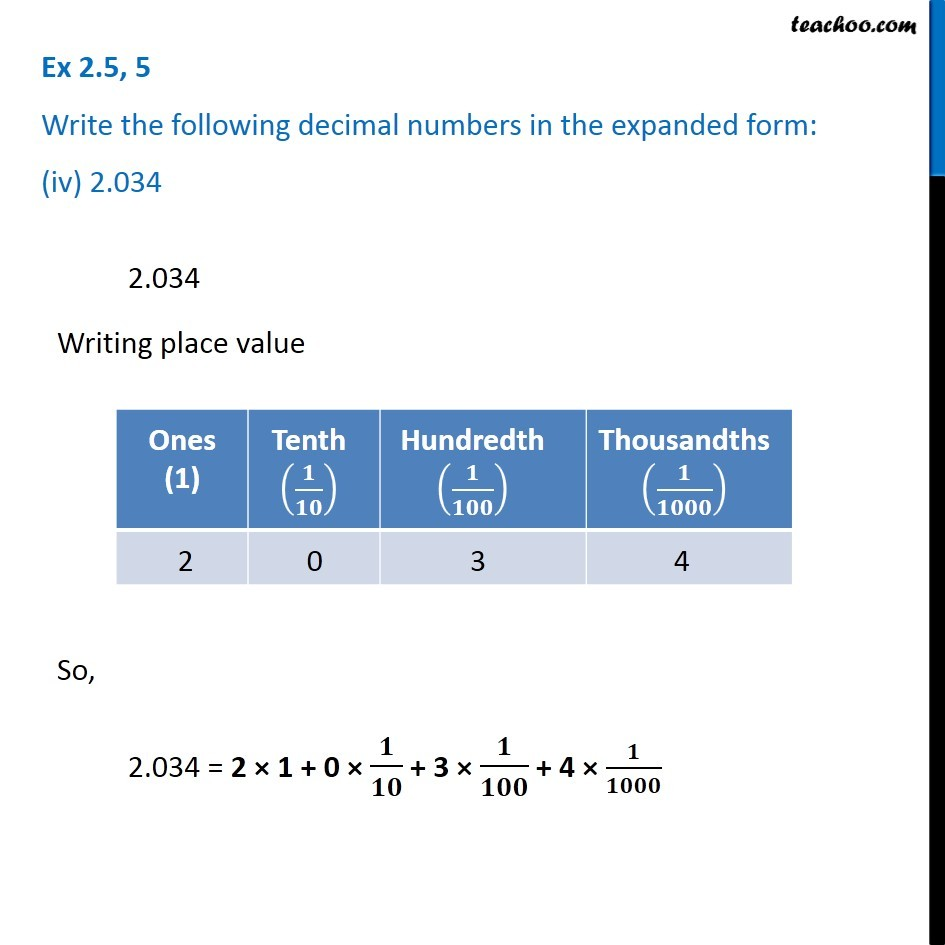Chapter 2 Class 7 Fractions and Decimals
Serial order wiseLearn in your speed, with individual attention - Teachoo Maths 1-on-1 Class

### Transcript

Question 5 Write the following decimal numbers in the expanded form: (i) 20.03 20.03 Writing place value So, 20.03 = 2 × 10 + 0 × 1 + 0 × 𝟏/𝟏𝟎 + 3 × 𝟏/𝟏𝟎𝟎 Question 5 Write the following decimal numbers in the expanded form: (ii) 2.03 2.03 Writing place value So, 2.03 = 2 × 1 + 0 × 𝟏/𝟏𝟎 + 3 × 𝟏/𝟏𝟎𝟎 Question 5 Write the following decimal numbers in the expanded form: (iii) 200.03 200.03 Writing place value So, 200.03 = 2 × 100 + 0 × 10 + 0 × 1 + 0 × 𝟏/𝟏𝟎 + 3 × 𝟏/𝟏𝟎𝟎 Question 5 Write the following decimal numbers in the expanded form: (iv) 2.034 2.034 Writing place value So, 2.034 = 2 × 1 + 0 × 𝟏/𝟏𝟎 + 3 × 𝟏/𝟏𝟎𝟎 + 4 × 𝟏/𝟏𝟎𝟎𝟎